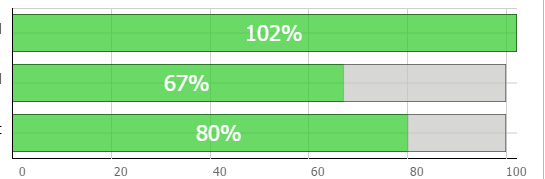# the X axis in a 3 element horiz chart gets skewed

#1

using stacked barH horiz bar chart that otherwise works, when I allow values over 100 (%) then the x axis values change

I had logic to clamp the value to 100 % but found it needs to reflect either the true value or have some way to show its past 100%. right now on one if the first charts value is 102? then the horiz x axis starts at -2 and ends at 98 ??

there are 3 bars it would be nice if it just scales as they all start at zero % and the number is right, so if we have 102, 80 and 67 it needs to always start the chart at 0 but at least end on some even number, or end on the 5’s 95/100/105/110/115 etc and not end at 98?

its a great control, but any ideas on this are greatly appreciated

#2

Hello,

could you please provide a sample code for the problem ?

#3

[code] myBarChart = new dhtmlXChart({
multiline: true,
view: “stackedBarH”,
container: “chartDiv”,
value: “#spent#”,

``````            label: function (obj) {

if (obj.rname == "Remaining" && timeused > 100 ) {
return "<span style='font-size:22px; color: red'>" + obj.spent + "%" + "</span>";
}
return "<span style='font-size:22px; color: white'>" + obj.spent + "%" + "</span>";
},
color: "#5ac923",
width: 38,

alpha: 0.7,
xAxis: {
start: 0,
step:20,

end: 100
},
padding: {
left: 250,
right: 18,
},
yAxis: {

template: function (obj) {

return "<p style='font-size:14px;color:black;'>" + obj.rname + "</p>" + "&nbsp;&nbsp;&nbsp;&nbsp;&nbsp;&nbsp;&nbsp;&nbsp;&nbsp;";
}
}
});

myBarChart.addSeries({
value: "#rrmain#",
color: "#c5c6c4",
label: ""
});
my_data = [{ spent: Remaining, rrmain: quotient1, rname: "Elapsed&nbsp;&nbsp;&nbsp;&nbsp;   " },
{ spent: Itemcompl, rrmain: quotient2, rname: "Estimate of Items Complete&nbsp;&nbsp;&nbsp;&nbsp;" },
{ spent: Moneyspent, rrmain: quotient3, rname: "Money Spent&nbsp;&nbsp;&nbsp;&nbsp;   " }];

myBarChart.parse(my_data, "json");[/code]``````

#4

the scaling problem comes from adding a 2nd series, which was in the requirement to show the chart value against a grey background the length of the chart

update: it still wont work. the logic to find the largest of the 3 values? is arbitrary. If the 3 values are 102, 87, 80 it may find any of the 3 to be the largest number. in one use with an SQL record it works but some other one fails. will try converting in the server/backend instead.

wrong version:

``` amtSpent = "40"; total = "100"; Remaining = "@Model.TimeElapsed"; quotient1 = (100 - Remaining); Itemcompl= "@Model.ItemsCompleted"; quotient2 = (100 - Itemcompl); Moneyspent = "@Model.MoneySpent"; quotient3 = (100 - Moneyspent); pSpent = (1 / (total / amtSpent)) * 100; perSpent = Math.round(pSpent); Overflowed = "@Model.ItemOverdue";```

Here the 2nd series is clamped to 0 if we’re over 100% Make the 2nd series 0 scales it right

``` amtSpent = "40"; total = "100"; Remaining = "@Model.TimeElapsed"; quotient1 = Remaining > 99? 0: (100 - Remaining); Itemcompl= "@Model.ItemsCompleted"; quotient2 = Itemcompl> 99? 0: (100 - Itemcompl); Moneyspent = "@Model.MoneySpent"; quotient3 = Moneyspent > 99? 0: (100 - Moneyspent); pSpent = (1 / (total / amtSpent)) * 100; perSpent = Math.round(pSpent); Overflowed = "@Model.ItemOverdue";```

#5note that the one with 80% no longer falls on the 80% line, this is unusable. the bottom 2, the grey area extends past 100%, this is wrong. The top one can go past.

I guess the fix was to find the largest of the 3 numbers and use this to scale the thing, largest value = “end” value. Was tricky because of how the logic seemed backwards at first:

`` biggest3 = biggest1 > biggest2 ? biggest2 : biggest1;``

seems like biggest 1 greater than biggest 2? yes? then use biggest 1, otherwise 2, reversed it and it discriminates right